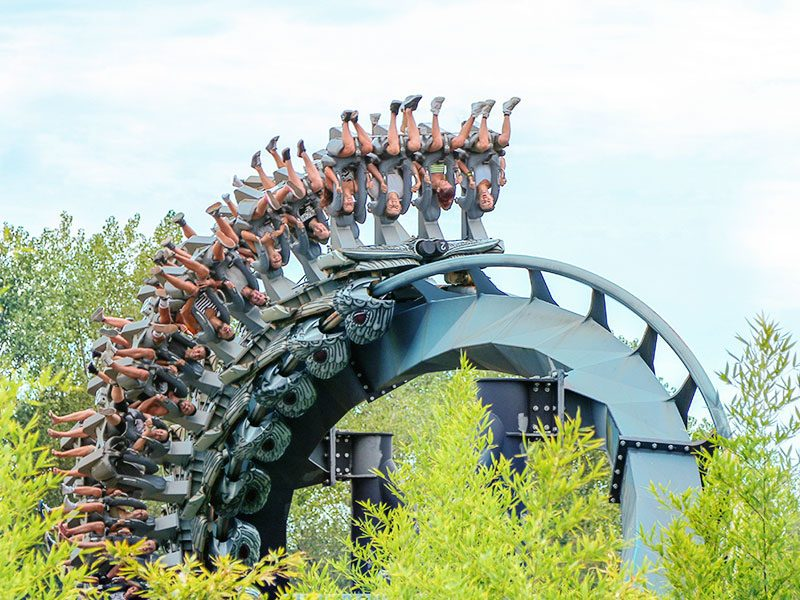# Matebilandia

An interdisciplinary course that analyses Mirabilandia’s attractions from a physical and mathematical perspective. Choose from one of 4 courses, subject to availability at the time of booking.

Project objectives:

• to give students a vision of physics and maths as tools for exploring the world around us, by making use of emotional involvement, a prerequisite for better learning;
• to carry out experiments not possible in school laboratories, and to experiment with the practical application and unexpectedly fun aspects of maths and physics  concepts;
• to bridge the gap between what you study in school and real life, between theory and practice, using real context such as the Park and its attractions.

AGE

DURATION

TEACHING MATERIAL

Upper secondary (14-18 years)
1 hour 50 mins
€5 per person
Worksheets and supporting materials for the activities

The activity includes a course of your choice (subject to availability) from  these 4 options:

## MODELLING OF KATUN. DISCOVERING THE MATHEMATICAL CURVES OF A ROLLERCOASTER

A mathematics course that observes the Katun inverted coaster, a veritable mine of mathematical curves, “with a mathematical eye”. We analyse curves, from those we are most familiar with, such as straight line and parabola, to the lesser known such as the clothoid loop, interesting and rich in practical applications.

Activities include:

• the study of the straight line: the angle of inclination on the ascent of the attraction is determined using different methods, and the data obtained are compared;
• the study of parabola; the profile of the attraction is observed, and we make hypotheses that are checked by using a mathematical machine to reconstruct the curve;
• analysing the characteristics of the Katun loop, and finding the mathematical curve that best describes its shape.

## KATUN. FROM REALITY TO MODEL: LAWS OF PHYSICS AND MATHEMATICAL CURVES

Maths and physics course that analyses the sensations experienced onboard the Katun inverted coaster, in the light of the laws of physics and curves that describe the trajectories.

Activities include:

• analysis of the sensations experienced onboard the ride due to forces in play;
• measurement of accelerations and variations in atmospheric pressure during motion;
• physical-mathematical analysis of the first descent: the parabolic motion in physics, research of the mathematical properties of the parabola and verification of the validity of the mathematical model for the description of the trajectory;
• analysis of the physical-mathematical characteristics of the Katun loop: acting forces, search for the mathematical curve that best describes its shape, comparison with circular trajectory.

## EUROWHEEL. A MATTER OF POINT OF VIEW: CONICS AND MOTIONS

The Ferris Wheel lends itself to a mathematical and physical analysis of the type of motion and profile, faced with various original approaches.

The activities carried out include:

• using different methods to measure the circumference of the Wheel. Comparison and evaluation of the methods used;
• analysis of the wheel’s motion: uniform circular motion and harmonic motion;
• reproduction of the attraction profile and reconstruction of the curve using mathematical machines and/or  of the conical parts (circumference, ellipse, parabola, hyperbola) using various models and machines.

## COLAZIONE DA PAPERE AND RAPTOTANA: MATHS AND PHYSICS IN ROTATION

Starting with the sensations experienced when subjected to rotations, the trajectories travelled are studied from a maths and physics viewpoint.

Activities include:

• measuring the characteristic quantities of motion;
• reproduction of the attraction’s movements and the exploration of trajectories using graphic calculators.

## INFORMATION AND BOOKING

The choice of course  is made according to availability at the time of telephone booking.

Parco della Standiana Srl - SS 16 Adriatica km. 162

Loc. Mirabilandia , 48125 Savio (RA) - Italy

Tel +39 0544 561156  Fax +39 0544 560195Subscribe now!